# Formulas tab in Sheets

Formulas tab in Sheets Windows

The Formulas dropdown includes all of the controls needed to manage your spreadsheet formulas effectively.More Functions – Contains a list of all the formulas available in OfficeSuite alongside detailed descriptions of their functionality: Common – Contains more commonly used formulas, like AVERAGE and SUM. All – Displays the full list of formulas currently available in OfficeSuite. Database – Contains database-related formulas such as maximum and minimum database values or extracting records from a database. Information – Informational formulas allow you to make adjustments based on cell conditions, such as whether cells contain even numbers, return errors, and others. Logical – Logical arguments are those that can manage values like true/false. Maths– Contains mathematical formulas, including trigonometric functions and logarithms. Date & Time – Lets you write formulas that operate based on date and time parameters. Statistical – Provides formulas found in statistics like standard deviation and frequency. Financial – Finance formulas include calculating interest payments and depreciation. Reference – Allows you to look up references and values of a cell range. Text – Text formulas offer options for capitalizing text entries, replacing characters, searching for entries, and more. Compatibility – Provides a wide selection of variance and distribution formulas.Define Name... – Lets you name a particular cell or cell range, its range, and which sheets make up its scope.Name Manager... – Displays all of the named cells and cell ranges in the spreadsheet.Recalculate – Forces the selected formula to be recalculated. Useful for applying changes made to updated formulas and cells.Auto Sum – Performs quick calculations of the numbers in a selected cell range and displays the result. Using this with only one cell selected will provide the sum of the above cell only.

Formulas tab in Sheets Android

Formulas are part of the core functionality of spreadsheets, and the Formulas tab includes all of the controls needed to effectively manage your formulas.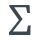Auto Sum – Performs quick calculations of the numbers in a selected cell range and displays the result. Using this with only one cell selected will allow you to enter cell ranges manually.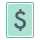Financial – Finance formulas include calculating interest payments and depreciation.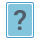Logical – Logical arguments are those that can manipulate values like true/false.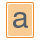Text – Text formulas offer options for capitalizing text entries, replacing characters, searching for entries, and more.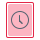Date & Time – Lets you write formulas that operate based on date and time parameters.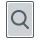Reference – Allows you to lookup references and values of a cell range.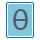Math – Contains mathematical formulas including trigonometric functions and logarithms.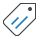Define Name – Lets you name a particular cell or cell range, its range, and which sheets make up its scope.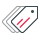Name Manager – Displays all of the named cells and cell ranges in the spreadsheet.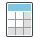Recalculate – Forces the selected formula to be recalculated. Useful for applying changes made to updated formulas and cells.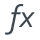Insert Function – Contains a list of all the formulas available in OfficeSuite alongside detailed descriptions of their functionality: Common – Contains more commonly used formulas, like AVERAGE and SUM. All – Displays the full list of formulas currently available in OfficeSuite. Math & Trig – Contains mathematical formulas including trigonometric functions and logarithms. Logical – Logical arguments are those that can manipulate values like true/false. Statistical – Provides formulas found in statistics like standard deviation and frequency. Text – Text formulas offer options for capitalizing text entries, replacing characters, searching for entries, and more. Reference – Allows you to lookup references and values of a cell range. Date & Time – Lets you write formulas that operate based on date and time parameters. Finance – Finance formulas include calculating interest payments and depreciation. Information – Informational formulas allow you to make adjustments based on cell conditions, such as whether cells contain even numbers, return errors, and others. Database – Contains database-related formulas such as maximum and minimum database values or extracting records from a database.

Formulas tab in Sheets iOS

Formulas are part of the core functionality of spreadsheets, and the Formulas tab includes all of the controls needed to effectively manage your formulas.Insert Function – Contains a list of all the formulas offered by OfficeSuite for iOS alongside detailed descriptions of their functionality: Common – Contains more commonly used formulas, like AVERAGE and SUM. All – Displays the full list of formulas currently available in OfficeSuite. Database – Contains database-related formulas such as maximum and minimum database values or extracting records from a database. Information – Informational formulas allow you to make adjustments based on cell conditions, such as whether cells contain even numbers, return errors, and others. Logical – Logical arguments are those that can manipulate values like true/false. Math – Contains mathematical formulas including trigonometric functions and logarithms. Date & Time – Lets you write formulas that operate based on date and time parameters. Statistical – Provides formulas found in statistics like standard deviation and frequency. Financial – Finance formulas include calculating interest payments and depreciation. Lookup – Allows you to lookup references and values of a cell range. Text – Text formulas offer options for capitalizing text entries, replacing characters, searching for entries, and more. Compatibility – Contains advanced mathematical conversions including binomial distribution and standard deviation.Define Name – Lets you name a particular cell or cell range, and which sheets make up its scope.Name Manager – Displays all of the named cells and cell ranges in the spreadsheet.Recalculate – Forces the selected formula to be recalculated. Useful for applying changes made to updated formulas and cells.Auto Sum – Performs quick calculations of the numbers in a selected cell range and displays the result: Sum – Adds up the numbers in the cell range. Average – Displays the average value of the numbers in the cell range. Count – Counts the number of cells in the range that contain numbers. Max – Displays the largest value of the numbers in the cell range. Min – Displays the smallest value of the numbers in the cell range. Median – Displays the middle number of the numbers in the cell range.Financial – Finance formulas include calculating interest payments and depreciation.Logical – Logical arguments are those that can manipulate values like true/false.Text – Text formulas offer options for capitalizing text entries, replacing characters, searching for entries, and more.Date & Time – Lets you write formulas that operate based on date and time parameters.Reference – Allows you to lookup references and values of a cell range.Math – Contains mathematical formulas including trigonometric functions and logarithms.
<% if (previousArticle || nextArticle) { %>
<% if (previousArticle) { %>
<% if (previousTitle) { %>

#### <%= previousTitle %>

<% } %> <%= previousArticle.title %>
<% } %> <% if (nextArticle) { %>
<% if (nextTitle) { %>

#### <%= nextTitle %>

<% } %> <%= nextArticle.title %>
<% } %>
<% } %>